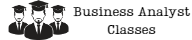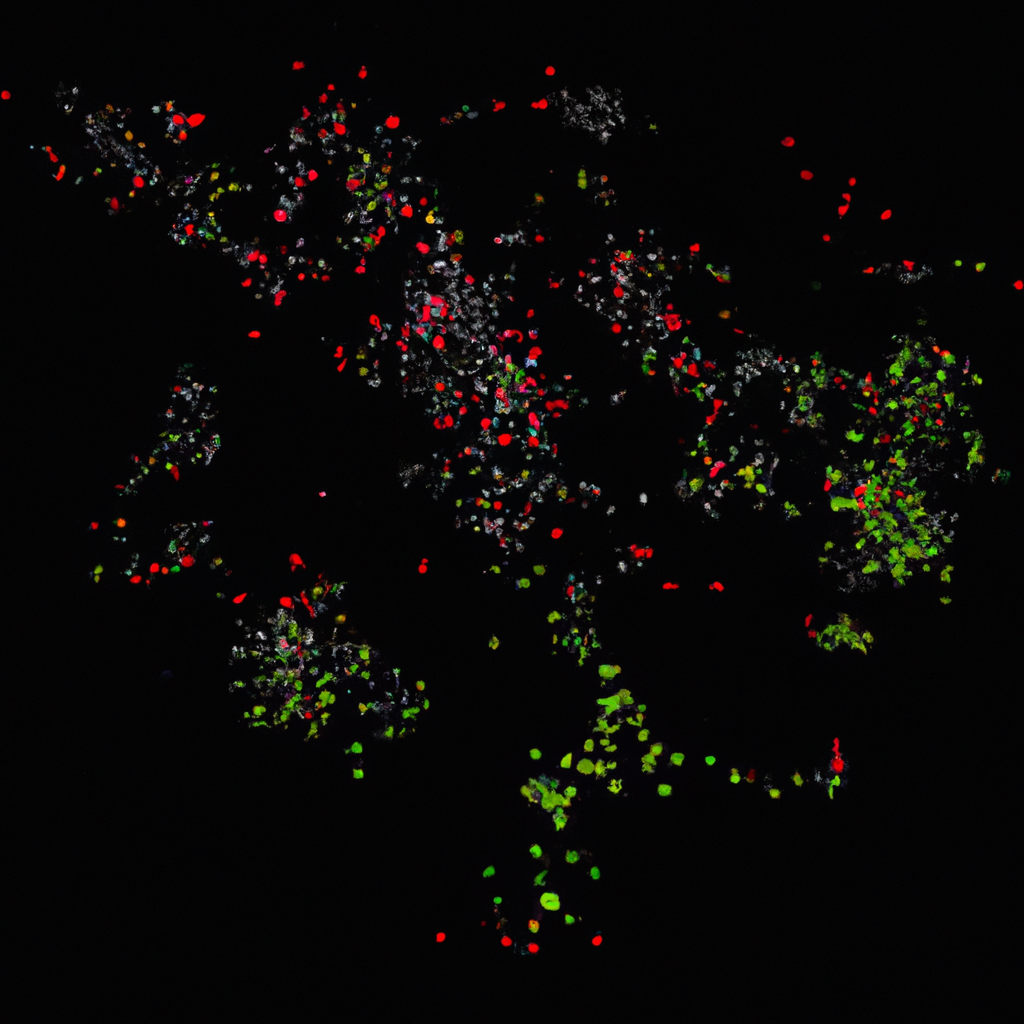Search

# How to Use Factor Analysis to Identify Underlying Dimensions in Data

As data scientists, we are tasked with analyzing large and complex data sets to extract meaningful insights that can help solve real-world problems. One of the most powerful tools in our arsenal is factor analysis. Factor analysis is a statistical method used to identify underlying dimensions in data and has applications in social sciences, psychology, marketing, and finance. In this article, we will explore the concept of factor analysis and how it can be used to uncover hidden patterns in data.## What is Factor Analysis?

Factor analysis is a statistical method used to identify underlying dimensions in a data set. It is a multivariate technique that aims to explain the correlation between observed variables by identifying a smaller number of unobserved variables, called factors. These factors are the underlying dimensions that influence the observed variables, and they represent the common variance among the observed variables. The goal of factor analysis is to reduce the number of observed variables to a smaller number of factors that can explain most of the variance in the data.

## Types of Factor Analysis

There are two main types of factor analysis: exploratory factor analysis (EFA) and confirmatory factor analysis (CFA).

### Exploratory Factor Analysis

Exploratory factor analysis is used to identify the underlying dimensions in a data set when we do not have any prior knowledge about the number of factors or the relationship between the observed variables and the factors. EFA is an iterative process that involves extracting the factors, rotating them to improve interpretability, and selecting the appropriate number of factors based on statistical criteria.

### Confirmatory Factor Analysis

Confirmatory factor analysis is used to test a theory or hypothesis about the relationship between the observed variables and the factors. CFA is a more structured approach, and the number of factors and their relationships with the observed variables are specified beforehand. CFA is used to confirm or reject a pre-specified factor structure based on the goodness of fit of the model to the data.

## Steps in Factor Analysis

The steps involved in factor analysis are as follows:

### Step 1: Data Preparation

The first step in factor analysis is to prepare the data. This involves checking for missing values, outliers, and normality of the data. The data should be cleaned and transformed if necessary to meet the assumptions of factor analysis.

### Step 2: Factor Extraction

The second step is to extract the factors. This involves using a statistical method to identify the factors that underlie the observed variables. The most commonly used method is principal component analysis (PCA), which extracts the factors that explain the most variance in the data.

### Step 3: Factor Rotation

The third step is to rotate the factors to improve interpretability. The goal of rotation is to transform the factors into a new set of uncorrelated factors that are easier to interpret. The most commonly used rotation method is orthogonal rotation, which produces uncorrelated factors.

### Step 4: Factor Selection

The fourth step is to select the appropriate number of factors. This involves using statistical criteria to determine the number of factors that should be retained. The most commonly used criteria are eigenvalues, scree plot, and parallel analysis.

### Step 5: Factor Interpretation

The fifth step is to interpret the factors. This involves looking at the factor loadings, which represent the correlation between the observed variables and the factors. High factor loadings indicate that the observed variables are strongly correlated with the factors, and vice versa. The factors should be named based on their interpretation, and their relationship with the observed variables should be explained.

## Applications of Factor Analysis

Factor analysis has many applications in different fields. Some of the common applications are:

### Social Sciences

Factor analysis is used in social sciences to identify the underlying dimensions of personality traits, attitudes, and behaviors. It is used to develop scales and questionnaires that measure these dimensions.

### Psychology

Factor analysis is used in psychology to identify the underlying dimensions of intelligence, cognitive abilities, and mental disorders. It is used to develop tests and assessments that measure these dimensions.

### Marketing

Factor analysis is used in marketing to identify the underlying dimensions of consumer behavior, such as brand loyalty, purchase intention, and product preferences. It is used to develop marketing strategies that target these dimensions.

### Finance

Factor analysis is used in finance to identify the underlying dimensions of risk and return in investment portfolios. It is used to develop asset allocation strategies that optimize risk-adjusted returns.

## Conclusion

Factor analysis is a powerful tool that can help extract meaningful insights from complex data sets. It is a multivariate technique that aims to identify the underlying dimensions that influence the observed variables. There are two main types of factor analysis: exploratory factor analysis and confirmatory factor analysis. The steps involved in factor analysis are data preparation, factor extraction, factor rotation, factor selection, and factor interpretation. Factor analysis has many applications in social sciences, psychology, marketing, and finance. By using factor analysis, we can uncover hidden patterns in data and make informed decisions based on the underlying dimensions that influence the observed variables.

### Try some of our classes

Enter your email and we’ll send you some samples of our favorite classes.

#### Course Categories

Quisque velit nisi, pretium ut lacinia in, elementum id enim.